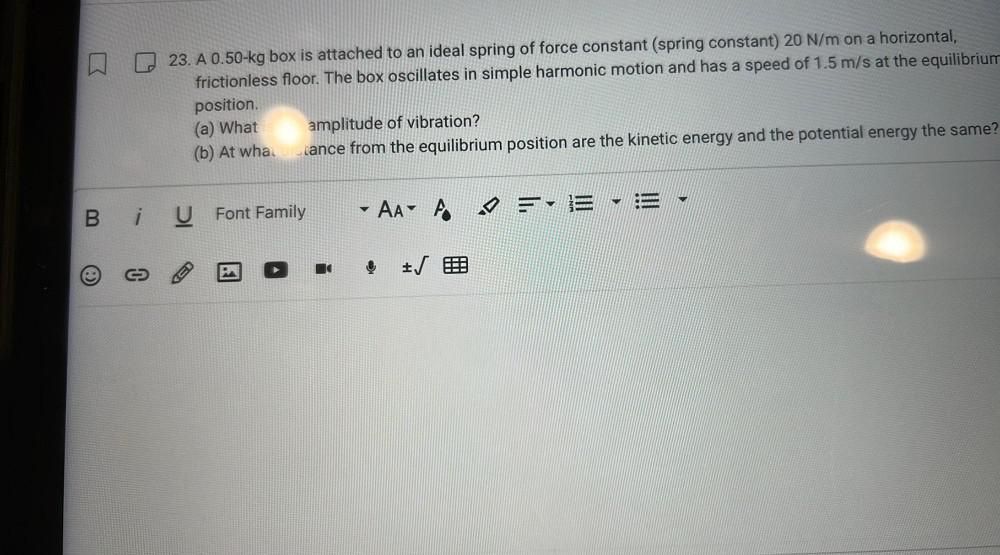Question:

# 23. A 0.50-kg box is attached to an ideal spring of force constant (spring constant) 20 N/m on a horizontal, frictionless floor.23. A 0.50-kg box is attached to an ideal spring of force constant (spring constant) 20 N/m on a horizontal, frictionless floor. The box oscillates in simple harmonic motion and has a speed of 1.5 m/s at the equilibrium position. (a) What amplitude of vibration? (b) At whal cance from the equilibrium position are the kinetic energy and the potential energy the same? B. i U Font Family Aa' A eV = E E IJ (